Would you like to see this cheatsheet in your native language? You can help us translating it on GitHub!

# States-based models with search optimization and MDP

By Afshine Amidi and Shervine Amidi

## Search optimization

In this section, we assume that by accomplishing action $a$ from state $s$, we deterministically arrive in state $\textrm{Succ}(s,a)$. The goal here is to determine a sequence of actions $(a_1,a_2,a_3,a_4,...)$ that starts from an initial state and leads to an end state. In order to solve this kind of problem, our objective will be to find the minimum cost path by using states-based models.

### Tree search

This category of states-based algorithms explores all possible states and actions. It is quite memory efficient, and is suitable for huge state spaces but the runtime can become exponential in the worst cases.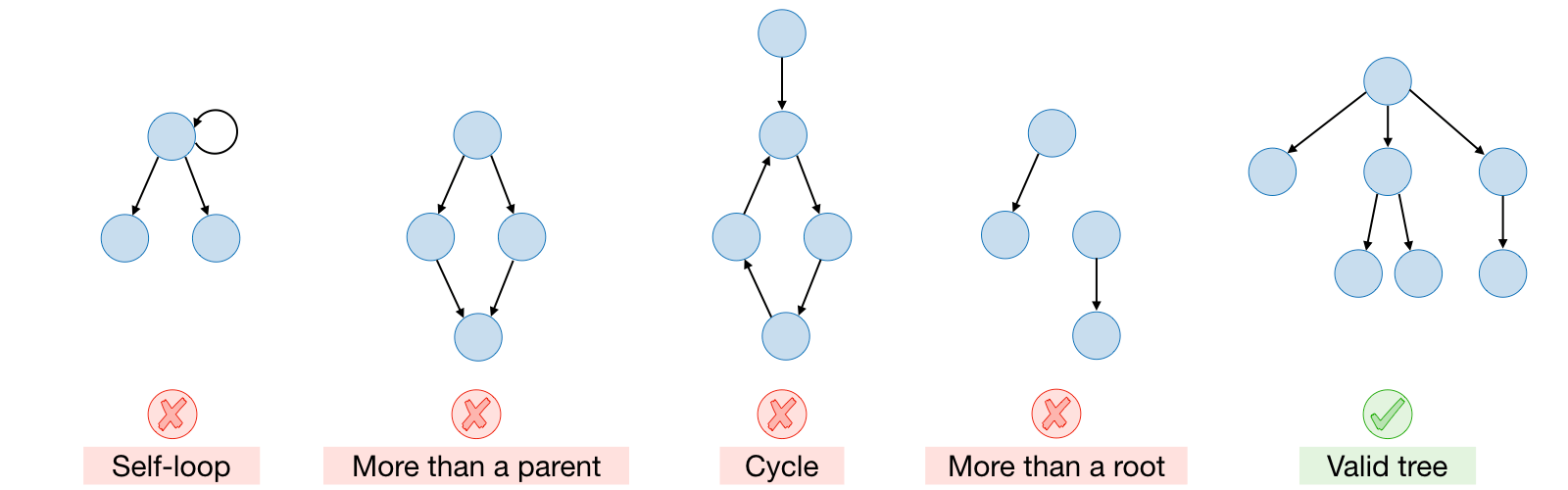Search problem ― A search problem is defined with:

• a starting state $s_{\textrm{start}}$
• possible actions $\textrm{Actions}(s)$ from state $s$
• action cost $\textrm{Cost}(s,a)$ from state $s$ with action $a$
• successor $\textrm{Succ}(s,a)$ of state $s$ after action $a$
• whether an end state was reached $\textrm{IsEnd}(s)$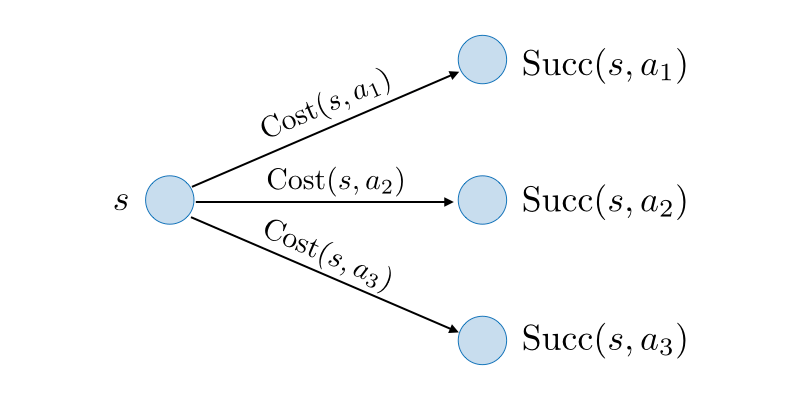The objective is to find a path that minimizes the cost.

Backtracking search ― Backtracking search is a naive recursive algorithm that tries all possibilities to find the minimum cost path. Here, action costs can be either positive or negative.

Breadth-first search (BFS) ― Breadth-first search is a graph search algorithm that does a level-by-level traversal. We can implement it iteratively with the help of a queue that stores at each step future nodes to be visited. For this algorithm, we can assume action costs to be equal to a constant $c\geqslant0$.
Depth-first search (DFS) ― Depth-first search is a search algorithm that traverses a graph by following each path as deep as it can. We can implement it recursively, or iteratively with the help of a stack that stores at each step future nodes to be visited. For this algorithm, action costs are assumed to be equal to 0.
Iterative deepening ― The iterative deepening trick is a modification of the depth-first search algorithm so that it stops after reaching a certain depth, which guarantees optimality when all action costs are equal. Here, we assume that action costs are equal to a constant $c\geqslant0$.

Tree search algorithms summary ― By noting $b$ the number of actions per state, $d$ the solution depth, and $D$ the maximum depth, we have:

 Algorithm Action costs Space Time Backtracking search any $\mathcal{O}(D)$ $\mathcal{O}(b^D)$ Breadth-first search $c\geqslant0$ $\mathcal{O}(b^d)$ $\mathcal{O}(b^d)$ Depth-first search 0 $\mathcal{O}(D)$ $\mathcal{O}(b^D)$ DFS-Iterative deepening $c\geqslant0$ $\mathcal{O}(d)$ $\mathcal{O}(b^d)$

### Graph search

This category of states-based algorithms aims at constructing optimal paths, enabling exponential savings. In this section, we will focus on dynamic programming and uniform cost search.

Graph ― A graph is comprised of a set of vertices $V$ (also called nodes) as well as a set of edges $E$ (also called links).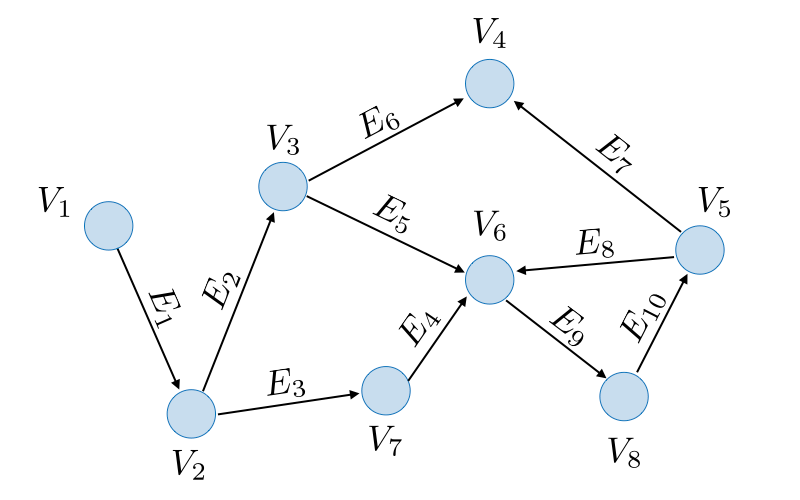Remark: a graph is said to be acylic when there is no cycle.

State ― A state is a summary of all past actions sufficient to choose future actions optimally.

Dynamic programming ― Dynamic programming (DP) is a backtracking search algorithm with memoization (i.e. partial results are saved) whose goal is to find a minimum cost path from state $s$ to an end state $s_\textrm{end}$. It can potentially have exponential savings compared to traditional graph search algorithms, and has the property to only work for acyclic graphs. For any given state $s$, the future cost is computed as follows:

$\boxed{\textrm{FutureCost}(s)=\left\{\begin{array}{lc}0 & \textrm{if IsEnd}(s)\\\underset{a\in\textrm{Actions}(s)}{\textrm{min}}\big[\textrm{Cost}(s,a)+\textrm{FutureCost(Succ}(s,a))\big] & \textrm{otherwise}\end{array}\right.}$
Remark: the figure above illustrates a bottom-to-top approach whereas the formula provides the intuition of a top-to-bottom problem resolution.

Types of states ― The table below presents the terminology when it comes to states in the context of uniform cost search:

 State Explanation Explored $\mathcal{E}$ States for which the optimal path has already been found Frontier $\mathcal{F}$ States seen for which we are still figuring out how to get there with the cheapest cost Unexplored $\mathcal{U}$ States not seen yet

Uniform cost search ― Uniform cost search (UCS) is a search algorithm that aims at finding the shortest path from a state $s_\textrm{start}$ to an end state $s_\textrm{end}$. It explores states $s$ in increasing order of $\textrm{PastCost}(s)$ and relies on the fact that all action costs are non-negative.
Remark 1: the UCS algorithm is logically equivalent to Dijkstra's algorithm.

Remark 2: the algorithm would not work for a problem with negative action costs, and adding a positive constant to make them non-negative would not solve the problem since this would end up being a different problem.

Correctness theorem ― When a state $s$ is popped from the frontier $\mathcal{F}$ and moved to explored set $\mathcal{E}$, its priority is equal to $\textrm{PastCost}(s)$ which is the minimum cost path from $s_\textrm{start}$ to $s$.

Graph search algorithms summary ― By noting $N$ the number of total states, $n$ of which are explored before the end state $s_\textrm{end}$, we have:

 Algorithm Acyclicity Costs Time/space Dynamic programming yes any $\mathcal{O}(N)$ Uniform cost search no $c\geqslant0$ $\mathcal{O}(n\log(n))$

Remark: the complexity countdown supposes the number of possible actions per state to be constant.

### Learning costs

Suppose we are not given the values of $\textrm{Cost}(s,a)$, we want to estimate these quantities from a training set of minimizing-cost-path sequence of actions $(a_1, a_2, ..., a_k)$.

Structured perceptron ― The structured perceptron is an algorithm aiming at iteratively learning the cost of each state-action pair. At each step, it:

• decreases the estimated cost of each state-action of the true minimizing path $y$ given by the training data,
• increases the estimated cost of each state-action of the current predicted path $y'$ inferred from the learned weights.

Remark: there are several versions of the algorithm, one of which simplifies the problem to only learning the cost of each action $a$, and the other parametrizes $\textrm{Cost}(s,a)$ to a feature vector of learnable weights.

### $\textrm{A}^{\star}$ search

Heuristic function ― A heuristic is a function $h$ over states $s$, where each $h(s)$ aims at estimating $\textrm{FutureCost}(s)$, the cost of the path from $s$ to $s_\textrm{end}$.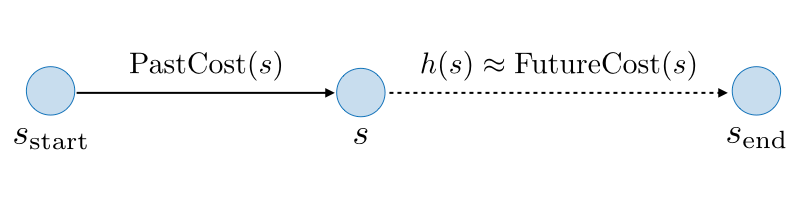Algorithm ― $A^{*}$ is a search algorithm that aims at finding the shortest path from a state $s$ to an end state $s_\textrm{end}$. It explores states $s$ in increasing order of $\textrm{PastCost}(s) + h(s)$. It is equivalent to a uniform cost search with edge costs $\textrm{Cost}'(s,a)$ given by:

$\boxed{\textrm{Cost}'(s,a)=\textrm{Cost}(s,a)+h(\textrm{Succ}(s,a))-h(s)}$

Remark: this algorithm can be seen as a biased version of UCS exploring states estimated to be closer to the end state.

Consistency ― A heuristic $h$ is said to be consistent if it satisfies the two following properties:

• For all states $s$ and actions $a$,
$\boxed{h(s) \leqslant \textrm{Cost}(s,a)+h(\textrm{Succ}(s,a))}$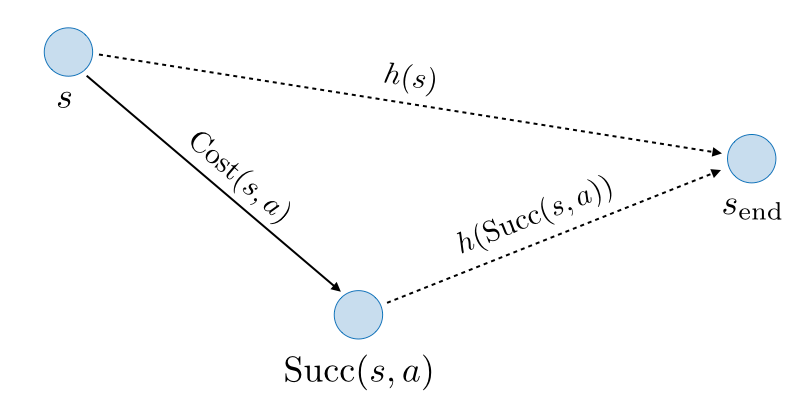• The end state verifies the following:
$\boxed{h(s_{\textrm{end}})=0}$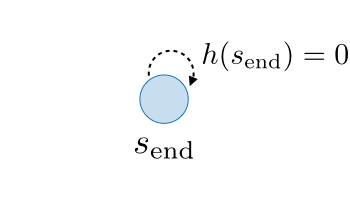Correctness ― If $h$ is consistent, then $A^*$ returns the minimum cost path.

Admissibility ― A heuristic $h$ is said to be admissible if we have:

$\boxed{h(s)\leqslant\textrm{FutureCost}(s)}$

Theorem ― Let $h(s)$ be a given heuristic. We have:

$\boxed{h(s)\textrm{ consistent}\Longrightarrow h(s)\textrm{ admissible}}$

Efficiency ― $A^*$ explores all states $s$ satisfying the following equation:

$\boxed{\textrm{PastCost}(s)\leqslant\textrm{PastCost}(s_{\textrm{end}})-h(s)}$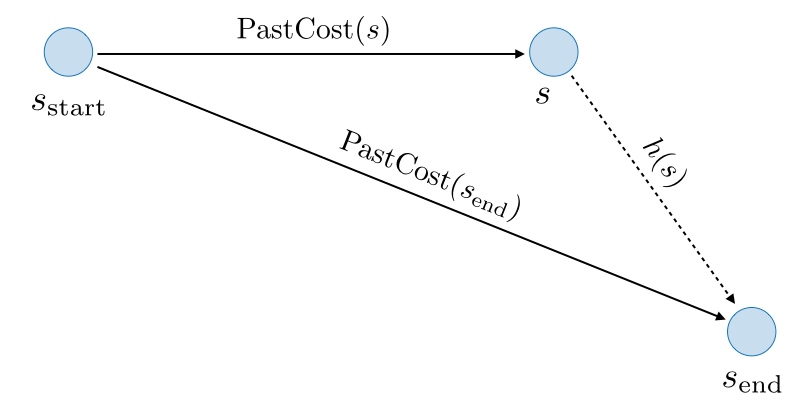Remark: larger values of $h(s)$ is better as this equation shows it will restrict the set of states $s$ going to be explored.

### Relaxation

It is a framework for producing consistent heuristics. The idea is to find closed-form reduced costs by removing constraints and use them as heuristics.

Relaxed search problem ― The relaxation of search problem $P$ with costs $\textrm{Cost}$ is noted $P_{\textrm{rel}}$ with costs $\textrm{Cost}_{\textrm{rel}}$, and satisfies the identity:

$\boxed{\textrm{Cost}_{\textrm{rel}}(s,a)\leqslant\textrm{Cost}(s,a)}$

Relaxed heuristic ― Given a relaxed search problem $P_{\textrm{rel}}$, we define the relaxed heuristic $h(s)=\textrm{FutureCost}_{\textrm{rel}}(s)$ as the minimum cost path from $s$ to an end state in the graph of costs $\textrm{Cost}_{\textrm{rel}}(s,a)$.

Consistency of relaxed heuristics ― Let $P_{\textrm{rel}}$ be a given relaxed problem. By theorem, we have:

$\boxed{h(s)=\textrm{FutureCost}_{\textrm{rel}}(s)\Longrightarrow h(s)\textrm{ consistent}}$

Tradeoff when choosing heuristic ― We have to balance two aspects in choosing a heuristic:

• Computational efficiency: $h(s)=\textrm{FutureCost}_{\textrm{rel}}(s)$ must be easy to compute. It has to produce a closed form, easier search and independent subproblems.
• Good enough approximation: the heuristic $h(s)$ should be close to $\textrm{FutureCost}(s)$ and we have thus to not remove too many constraints.

Max heuristic ― Let $h_1(s)$, $h_2(s)$ be two heuristics. We have the following property:

$\boxed{h_1(s),\textrm{ }h_2(s)\textrm{ consistent}\Longrightarrow h(s)=\max\{h_1(s),\textrm{ }h_2(s)\}\textrm{ consistent}}$

## Markov decision processes

In this section, we assume that performing action $a$ from state $s$ can lead to several states $s_1',s_2',...$ in a probabilistic manner. In order to find our way between an initial state and an end state, our objective will be to find the maximum value policy by using Markov decision processes that help us cope with randomness and uncertainty.

### Notations

Definition ― The objective of a Markov decision process is to maximize rewards. It is defined with:

• a starting state $s_{\textrm{start}}$
• possible actions $\textrm{Actions}(s)$ from state $s$
• transition probabilities $T(s,a,s')$ from $s$ to $s'$ with action $a$
• rewards $\textrm{Reward}(s,a,s')$ from $s$ to $s'$ with action $a$
• whether an end state was reached $\textrm{IsEnd}(s)$
• a discount factor $0\leqslant\gamma\leqslant1$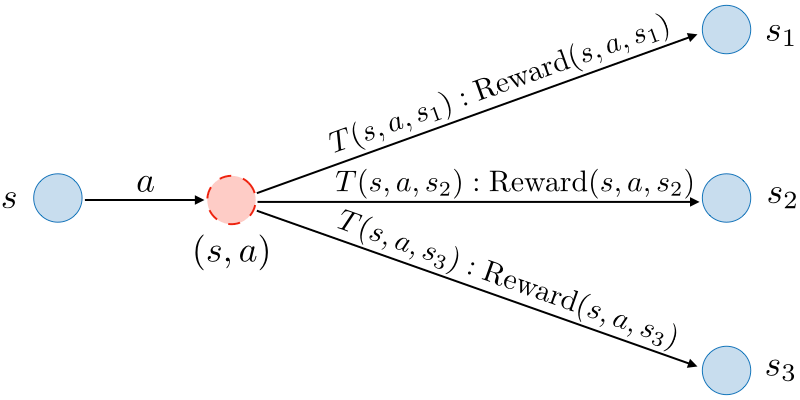Transition probabilities ― The transition probability $T(s,a,s')$ specifies the probability of going to state $s'$ after action $a$ is taken in state $s$. Each $s' \mapsto T(s,a,s')$ is a probability distribution, which means that:

$\forall s,a,\quad\boxed{\displaystyle\sum_{s'\in\textrm{ States}}T(s,a,s')=1}$

Policy ― A policy $\pi$ is a function that maps each state $s$ to an action $a$, i.e.

$\boxed{\pi : s \mapsto a}$

Utility ― The utility of a path $(s_0, ..., s_k)$ is the discounted sum of the rewards on that path. In other words,

$\boxed{u(s_0,...,s_k)=\sum_{i=1}^{k}r_i\gamma^{i-1}}$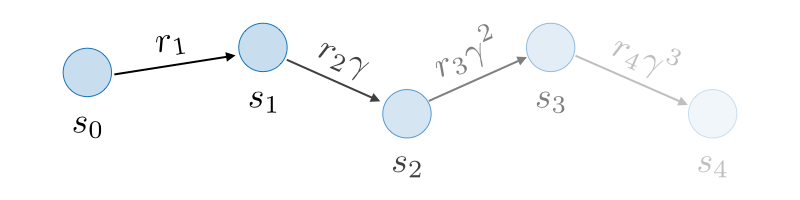The figure above is an illustration of the case $k=4$.

Q-value ― The $Q$-value of a policy $\pi$ at state $s$ with action $a$, also noted $Q_{\pi}(s,a)$, is the expected utility from state $s$ after taking action $a$ and then following policy $\pi$. It is defined as follows:

$\boxed{Q_{\pi}(s,a)=\sum_{s'\in\textrm{ States}}T(s,a,s')\left[\textrm{Reward}(s,a,s')+\gamma V_\pi(s')\right]}$

Value of a policy ― The value of a policy $\pi$ from state $s$, also noted $V_{\pi}(s)$, is the expected utility by following policy $\pi$ from state $s$ over random paths. It is defined as follows:

$\boxed{V_\pi(s)=Q_\pi(s,\pi(s))}$

Remark: $V_\pi(s)$ is equal to 0 if $s$ is an end state.

### Applications

Policy evaluation ― Given a policy $\pi$, policy evaluation is an iterative algorithm that aims at estimating $V_\pi$. It is done as follows:

• Initialization: for all states $s$, we have
$\boxed{V_\pi^{(0)}(s)\longleftarrow0}$
• Iteration: for $t$ from 1 to $T_{\textrm{PE}}$, we have
$\forall s,\quad\boxed{V_\pi^{(t)}(s)\longleftarrow Q_\pi^{(t-1)}(s,\pi(s))}$
with
$\boxed{Q_\pi^{(t-1)}(s,\pi(s))=\sum_{s'\in\textrm{ States}}T(s,\pi(s),s')\Big[\textrm{Reward}(s,\pi(s),s')+\gamma V_\pi^{(t-1)}(s')\Big]}$

Remark: by noting $S$ the number of states, $A$ the number of actions per state, $S'$ the number of successors and $T$ the number of iterations, then the time complexity is of $\mathcal{O}(T_{\textrm{PE}}SS')$.

Optimal Q-value ― The optimal $Q$-value $Q_{\textrm{opt}}(s,a)$ of state $s$ with action $a$ is defined to be the maximum $Q$-value attained by any policy. It is computed as follows:

$\boxed{Q_{\textrm{opt}}(s,a)=\sum_{s'\in\textrm{ States}}T(s,a,s')\left[\textrm{Reward}(s,a,s')+\gamma V_\textrm{opt}(s')\right]}$

Optimal value ― The optimal value $V_{\textrm{opt}}(s)$ of state $s$ is defined as being the maximum value attained by any policy. It is computed as follows:

$\boxed{V_{\textrm{opt}}(s)=\underset{a\in\textrm{ Actions}(s)}{\textrm{max}}Q_\textrm{opt}(s,a)}$

Optimal policy ― The optimal policy $\pi_{\textrm{opt}}$ is defined as being the policy that leads to the optimal values. It is defined by:

$\forall s,\quad\boxed{\pi_{\textrm{opt}}(s)=\underset{a\in\textrm{ Actions}(s)}{\textrm{argmax}}Q_\textrm{opt}(s,a)}$

Value iteration ― Value iteration is an algorithm that finds the optimal value $V_{\textrm{opt}}$ as well as the optimal policy $\pi_{\textrm{opt}}$. It is done as follows:

• Initialization: for all states $s$, we have
$\boxed{V_{\textrm{opt}}^{(0)}(s)\longleftarrow0}$
• Iteration: for $t$ from 1 to $T_{\textrm{VI}}$, we have
$\forall s,\quad\boxed{V_\textrm{opt}^{(t)}(s)\longleftarrow \underset{a\in\textrm{ Actions}(s)}{\textrm{max}}Q_\textrm{opt}^{(t-1)}(s,a)}$
with
$\boxed{Q_\textrm{opt}^{(t-1)}(s,a)=\sum_{s'\in\textrm{ States}}T(s,a,s')\Big[\textrm{Reward}(s,a,s')+\gamma V_\textrm{opt}^{(t-1)}(s')\Big]}$

Remark: if we have either $\gamma < 1$ or the MDP graph being acyclic, then the value iteration algorithm is guaranteed to converge to the correct answer.

### When unknown transitions and rewards

Now, let's assume that the transition probabilities and the rewards are unknown.

Model-based Monte Carlo ― The model-based Monte Carlo method aims at estimating $T(s,a,s')$ and $\textrm{Reward}(s,a,s')$ using Monte Carlo simulation with:

$\boxed{\widehat{T}(s,a,s')=\frac{\#\textrm{ times }(s,a,s')\textrm{ occurs}}{\#\textrm{ times }(s,a)\textrm{ occurs}}}$
and
$\boxed{\widehat{\textrm{Reward}}(s,a,s')=r\textrm{ in }(s,a,r,s')}$
These estimations will be then used to deduce $Q$-values, including $Q_\pi$ and $Q_\textrm{opt}.$

Remark: model-based Monte Carlo is said to be off-policy, because the estimation does not depend on the exact policy.

Model-free Monte Carlo ― The model-free Monte Carlo method aims at directly estimating $Q_{\pi}$, as follows:

$\boxed{\widehat{Q}_\pi(s,a)=\textrm{average of }u_t\textrm{ where }s_{t-1}=s, a_t=a}$
where $u_t$ denotes the utility starting at step $t$ of a given episode.

Remark: model-free Monte Carlo is said to be on-policy, because the estimated value is dependent on the policy $\pi$ used to generate the data.

Equivalent formulation - By introducing the constant $\eta=\frac{1}{1+(\#\textrm{updates to }(s,a))}$ and for each $(s,a,u)$ of the training set, the update rule of model-free Monte Carlo has a convex combination formulation:

$\boxed{\widehat{Q}_\pi(s,a)\leftarrow(1-\eta)\widehat{Q}_\pi(s,a)+\eta u}$
as well as a stochastic gradient formulation:
$\boxed{\widehat{Q}_\pi(s,a)\leftarrow\widehat{Q}_\pi(s,a) - \eta (\widehat{Q}_\pi(s,a) - u)}$

SARSA ― State-action-reward-state-action (SARSA) is a boostrapping method estimating $Q_\pi$ by using both raw data and estimates as part of the update rule. For each $(s,a,r,s',a')$, we have:

$\boxed{\widehat{Q}_\pi(s,a)\longleftarrow(1-\eta)\widehat{Q}_\pi(s,a)+\eta\Big[r+\gamma\widehat{Q}_\pi(s',a')\Big]}$

Remark: the SARSA estimate is updated on the fly as opposed to the model-free Monte Carlo one where the estimate can only be updated at the end of the episode.

Q-learning ― $Q$-learning is an off-policy algorithm that produces an estimate for $Q_\textrm{opt}$. On each $(s,a,r,s',a')$, we have:

$\boxed{\widehat{Q}_{\textrm{opt}}(s,a)\leftarrow(1-\eta)\widehat{Q}_{\textrm{opt}}(s,a)+\eta\Big[r+\gamma\underset{a'\in\textrm{ Actions}(s')}{\textrm{max}}\widehat{Q}_{\textrm{opt}}(s',a')\Big]}$

Epsilon-greedy ― The epsilon-greedy policy is an algorithm that balances exploration with probability $\epsilon$ and exploitation with probability $1-\epsilon$. For a given state $s$, the policy $\pi_{\textrm{act}}$ is computed as follows:

$\boxed{\pi_\textrm{act}(s)=\left\{\begin{array}{ll}\underset{a\in\textrm{ Actions}}{\textrm{argmax }}\widehat{Q}_\textrm{opt}(s,a) & \textrm{with proba }1-\epsilon\\\textrm{random from Actions}(s) & \textrm{with proba }\epsilon\end{array}\right.}$

## Game playing

In games (e.g. chess, backgammon, Go), other agents are present and need to be taken into account when constructing our policy.

Game tree ― A game tree is a tree that describes the possibilities of a game. In particular, each node is a decision point for a player and each root-to-leaf path is a possible outcome of the game.

Two-player zero-sum game ― It is a game where each state is fully observed and such that players take turns. It is defined with:

• a starting state $s_{\textrm{start}}$
• possible actions $\textrm{Actions}(s)$ from state $s$
• successors $\textrm{Succ}(s,a)$ from states $s$ with actions $a$
• whether an end state was reached $\textrm{IsEnd}(s)$
• the agent's utility $\textrm{Utility}(s)$ at end state $s$
• the player $\textrm{Player}(s)$ who controls state $s$

Remark: we will assume that the utility of the agent has the opposite sign of the one of the opponent.

Types of policies ― There are two types of policies:

• Deterministic policies, noted $\pi_p(s)$, which are actions that player $p$ takes in state $s$.
• Stochastic policies, noted $\pi_p(s,a)\in[0,1]$, which are probabilities that player $p$ takes action $a$ in state $s$.

Expectimax ― For a given state $s$, the expectimax value $V_{\textrm{exptmax}}(s)$ is the maximum expected utility of any agent policy when playing with respect to a fixed and known opponent policy $\pi_{\textrm{opp}}$. It is computed as follows:

$\boxed{V_{\textrm{exptmax}}(s)=\left\{\begin{array}{ll}\textrm{Utility}(s) & \textrm{IsEnd}(s)\\\underset{a\in\textrm{Actions}(s)}{\textrm{max}}V_{\textrm{exptmax}}(\textrm{Succ}(s,a)) & \textrm{Player}(s)=\textrm{agent}\\\displaystyle\sum_{a\in\textrm{Actions}(s)}\pi_{\textrm{opp}}(s,a)V_{\textrm{exptmax}}(\textrm{Succ}(s,a)) & \textrm{Player}(s)=\textrm{opp}\end{array}\right.}$

Remark: expectimax is the analog of value iteration for MDPs.
Minimax ― The goal of minimax policies is to find an optimal policy against an adversary by assuming the worst case, i.e. that the opponent is doing everything to minimize the agent's utility. It is done as follows:

$\boxed{V_{\textrm{minimax}}(s)=\left\{\begin{array}{ll}\textrm{Utility}(s) & \textrm{IsEnd}(s)\\\underset{a\in\textrm{Actions}(s)}{\textrm{max}}V_{\textrm{minimax}}(\textrm{Succ}(s,a)) & \textrm{Player}(s)=\textrm{agent}\\\underset{a\in\textrm{Actions}(s)}{\textrm{min}}V_{\textrm{minimax}}(\textrm{Succ}(s,a)) & \textrm{Player}(s)=\textrm{opp}\end{array}\right.}$

Remark: we can extract $\pi_{\textrm{max}}$ and $\pi_{\textrm{min}}$ from the minimax value $V_{\textrm{minimax}}$.
Minimax properties ― By noting $V$ the value function, there are 3 properties around minimax to have in mind:

• Property 1: if the agent were to change its policy to any $\pi_{\textrm{agent}}$, then the agent would be no better off.
$\boxed{\forall \pi_{\textrm{agent}},\quad V(\pi_{\textrm{max}},\pi_{\textrm{min}})\geqslant V(\pi_{\textrm{agent}},\pi_{\textrm{min}})}$
• Property 2: if the opponent changes its policy from $\pi_{\textrm{min}}$ to $\pi_{\textrm{opp}}$, then he will be no better off.
$\boxed{\forall \pi_{\textrm{opp}},\quad V(\pi_{\textrm{max}},\pi_{\textrm{min}})\leqslant V(\pi_{\textrm{max}},\pi_{\textrm{opp}})}$
• Property 3: if the opponent is known to be not playing the adversarial policy, then the minimax policy might not be optimal for the agent.
$\boxed{\forall \pi,\quad V(\pi_{\textrm{max}},\pi)\leqslant V(\pi_{\textrm{exptmax}},\pi)}$
In the end, we have the following relationship:
$\boxed{V(\pi_{\textrm{exptmax}},\pi_{\textrm{min}})\leqslant V(\pi_{\textrm{max}},\pi_{\textrm{min}})\leqslant V(\pi_{\textrm{max}},\pi)\leqslant V(\pi_{\textrm{exptmax}},\pi)}$

### Speeding up minimax

Evaluation function ― An evaluation function is a domain-specific and approximate estimate of the value $V_{\textrm{minimax}}(s)$. It is noted $\textrm{Eval}(s)$.

Remark: $\textrm{FutureCost}(s)$ is an analogy for search problems.

Alpha-beta pruning ― Alpha-beta pruning is a domain-general exact method optimizing the minimax algorithm by avoiding the unnecessary exploration of parts of the game tree. To do so, each player keeps track of the best value they can hope for (stored in $\alpha$ for the maximizing player and in $\beta$ for the minimizing player). At a given step, the condition $\beta < \alpha$ means that the optimal path is not going to be in the current branch as the earlier player had a better option at their disposal.
TD learning ― Temporal difference (TD) learning is used when we don't know the transitions/rewards. The value is based on exploration policy. To be able to use it, we need to know rules of the game $\textrm{Succ}(s,a)$. For each $(s,a,r,s')$, the update is done as follows:

$\boxed{w\longleftarrow w-\eta\big[V(s,w)-(r+\gamma V(s',w))\big]\nabla_wV(s,w)}$

### Simultaneous games

This is the contrary of turn-based games, where there is no ordering on the player's moves.

Single-move simultaneous game ― Let there be two players $A$ and $B$, with given possible actions. We note $V(a,b)$ to be $A$'s utility if $A$ chooses action $a$, $B$ chooses action $b$. $V$ is called the payoff matrix.

Strategies ― There are two main types of strategies:

• A pure strategy is a single action:
$\boxed{a\in\textrm{Actions}}$
• A mixed strategy is a probability distribution over actions:
$\forall a\in\textrm{Actions},\quad\boxed{0\leqslant\pi(a)\leqslant1}$

Game evaluation ― The value of the game $V(\pi_A,\pi_B)$ when player $A$ follows $\pi_A$ and player $B$ follows $\pi_B$ is such that:

$\boxed{V(\pi_A,\pi_B)=\sum_{a,b}\pi_A(a)\pi_B(b)V(a,b)}$

Minimax theorem ― By noting $\pi_A,\pi_B$ ranging over mixed strategies, for every simultaneous two-player zero-sum game with a finite number of actions, we have:

$\boxed{\max_{\pi_A}\min_{\pi_B}V(\pi_A,\pi_B)=\min_{\pi_B}\max_{\pi_A}V(\pi_A,\pi_B)}$

### Non-zero-sum games

Payoff matrix ― We define $V_p(\pi_A,\pi_B)$ to be the utility for player $p$.

Nash equilibrium ― A Nash equilibrium is $(\pi_A^*,\pi_B^*)$ such that no player has an incentive to change its strategy. We have:

$\boxed{\forall \pi_A, V_A(\pi_A^*,\pi_B^*)\geqslant V_A(\pi_A,\pi_B^*)}\quad\textrm{and}\quad\boxed{\forall \pi_B, V_B(\pi_A^*,\pi_B^*)\geqslant V_B(\pi_A^*,\pi_B)}$

Remark: in any finite-player game with finite number of actions, there exists at least one Nash equilibrium.